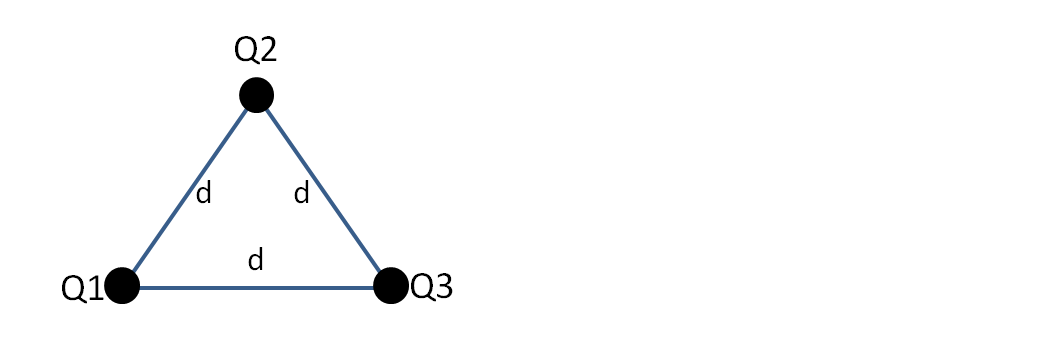Problem: Three identical point charges, Q = 3μC, are placed at the vertices of an equilateral triangle as shown in the figure. The length of each side of the triangle is d = 0.15m. Determine the magnitude and direction of the total electrostatic force on the charge at the top of the triangle.

99% (6 ratings)
Problem Details

Three identical point charges, Q = 3μC, are placed at the vertices of an equilateral triangle as shown in the figure. The length of each side of the triangle is d = 0.15m. Determine the magnitude and direction of the total electrostatic force on the charge at the top of the triangle.